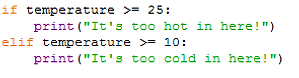top of page

## Mathematical Operators

When comparing two values, like in an if statement, there are a number of operators than can be used:In the example below the print line will only be executed if the temperature value is greater than 25:

If we entered 25 then the print line beneath would not run because is not above 25.

Alternatively, you can  use the greater than or equal to sign ( >= ) :You can use the less than ( < ) and less than or equal to sign ( <= ) in a similar way (and implement an elif at the same time):Now there’s only one thing missing from this program – we need an else statement for any value that is inputted between:Can you work out which sentences would be printed if we entered 8, 10 and 24?

The opposite of equal to ( == ) is not equal to ( != ). For example:A school is putting on a quiz for sixth form students.

Students enter their age and they will receive a ticket to the quiz if it is between (and including) 16 to 18.

Example solution:Embedded Python Editor